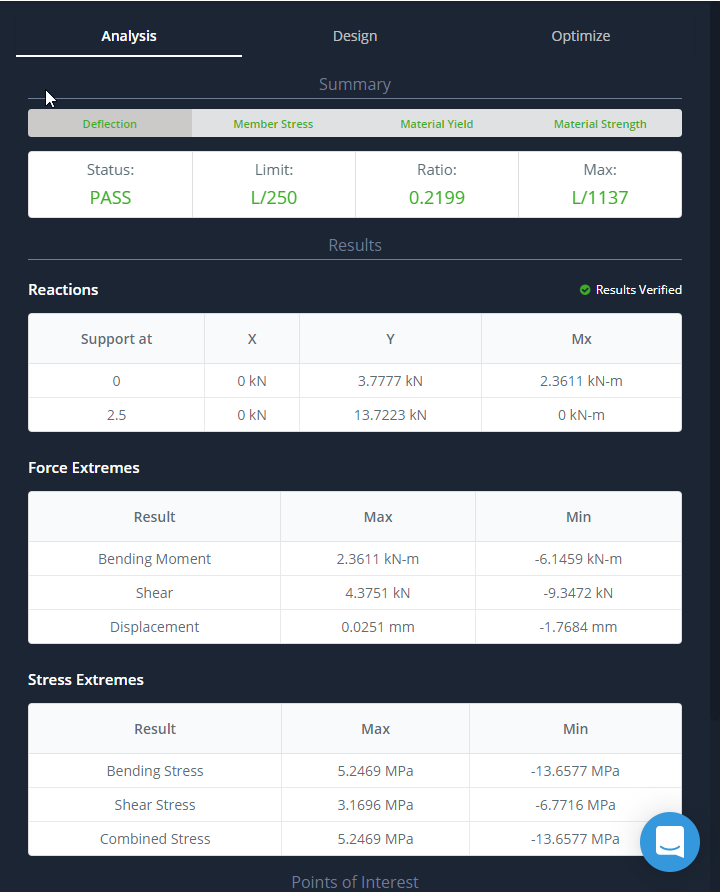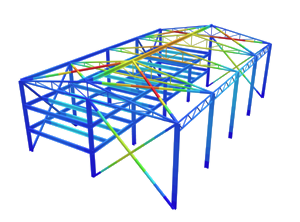SkyCiv Documentation

Your guide to SkyCiv software - tutorials, how-to guides and technical articles

1. Home
2. SkyCiv Beam
3. Interpreting Results

# Interpreting Results

## Interpreting the reactions, bending moment, shear, deflection and stresses results from SkyCiv Beam

When you’ve finished building your model, you can solve for various results by clicking the green ‘Solve’ button located to the top right of your model. The model below continues the example from the previous sections.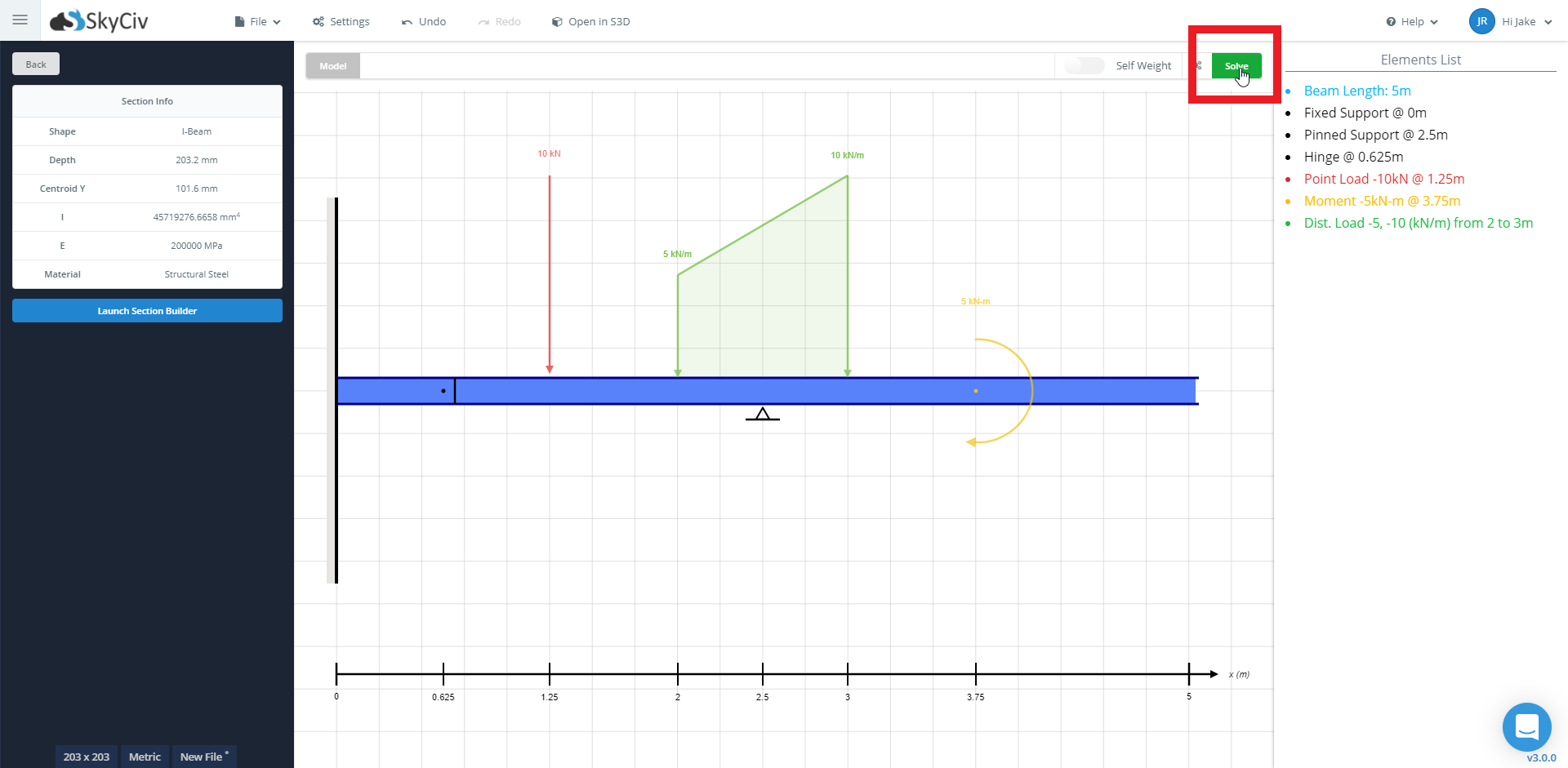Clicking the solve button will bring you to the results page where you access analysis results. The top menu allows you to toggle between individual results or return to your model. Using the left panel, you can access results such as:

• Reactions at Supports / Free Body Diagram
• Bending Moment Diagram
• Shear Force Diagram
• Deflection
• Bending Stress
• Shear Stress
• Beam Section
• 3D Rendering

Furthermore, using the right-side analysis tab you can access detailed results such as

• Quick Results Summary
• Reaction Values (X, Y Mx) at each support
• Force Extremes
• Stress Extremes
• Points of Interest Table

Note: To access all results including those related to stress, deflection, and 3D rendering, a section is required to be added from the section builder to your beam model.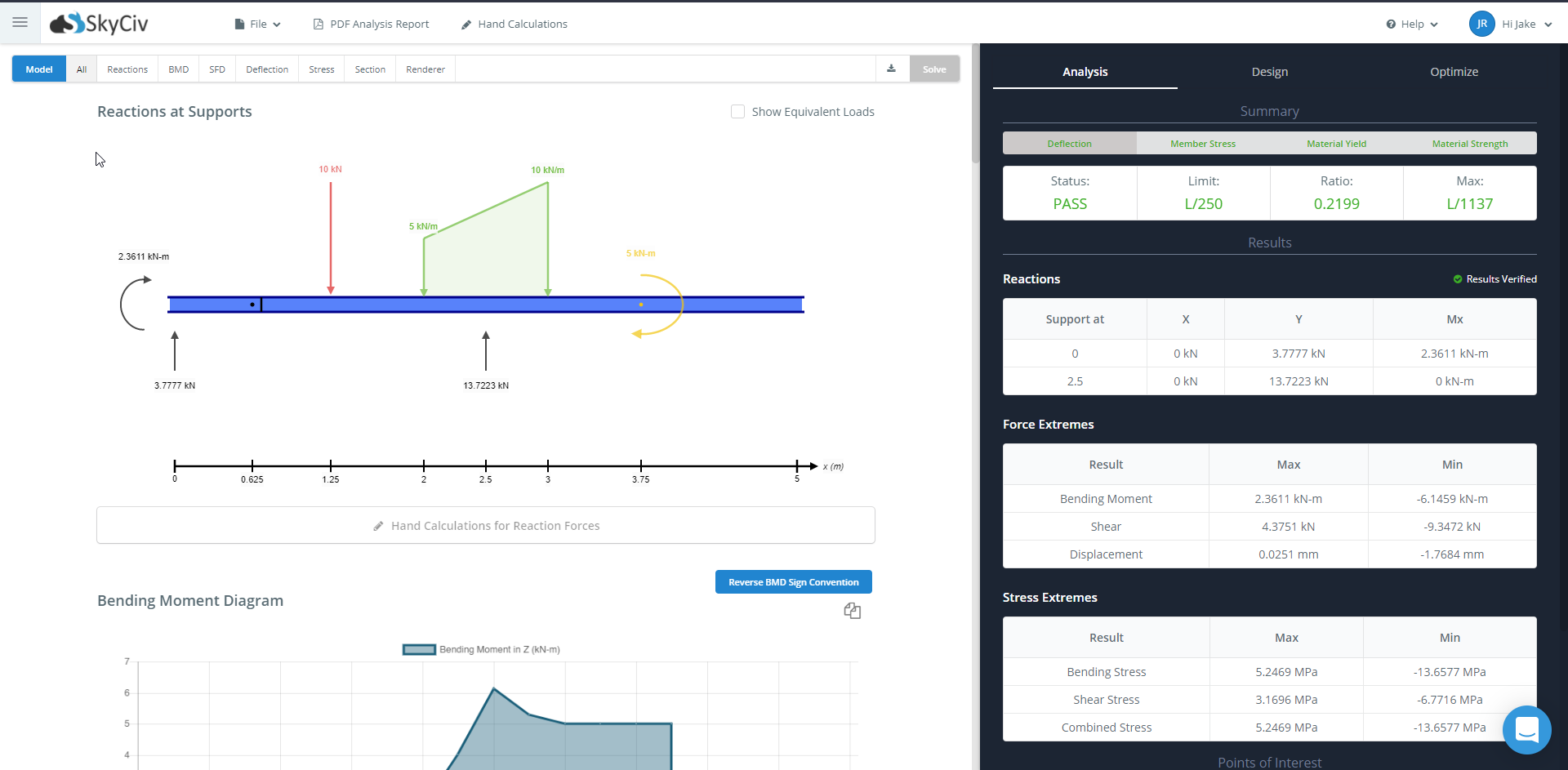## Free Body Diagram (FBD)

A free body diagram is available to view the reactions at the supports of the model. We also provide hand calculations to demonstrate how the reaction results were calculated in a step-by-step manner. More details about the Hand Calculations are provided in its own section.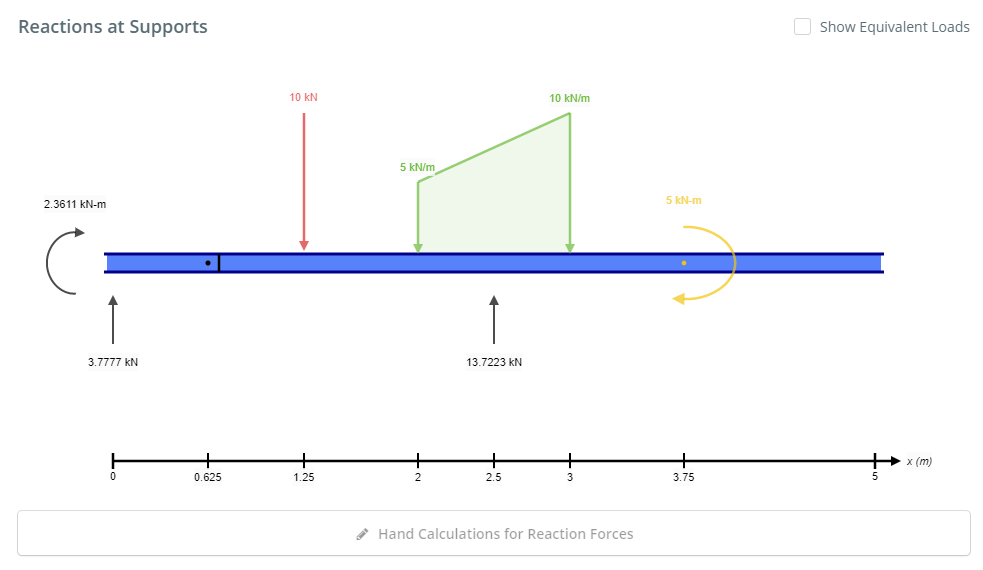## Beam Section

A summary of the section used for the beam is presented with the results.  The geometry of the beam together with its dimensions is displayed on the left. To the right, tables are presented to display the geometric, bending, shear, and torsion properties. At the bottom, the material properties are summarised in the table.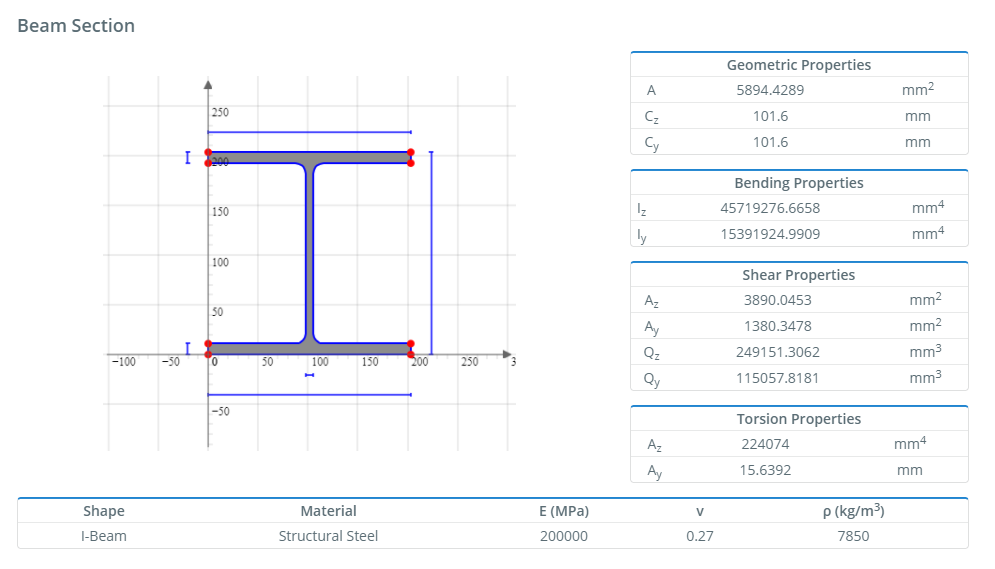## Shear Force Diagram (SFD)

The Shear Force Diagram (SFD) of your model is presented with the results. As expected, it plots the shear force values against the length of the beam.

At the bottom of the SFD, the Shear Force Equations are presented at each discontinuity. Use the range after the equation to match the equation to its discontinuity on the graph.

To find the value at a specific point on the SFD, use to POI table on the left or hover over the graph to get an exact value at that point.

Hand calculations are also available for the SFD to demonstrate how the Shear Force Equations were derived. More details about the Hand Calculations are provided in its own section.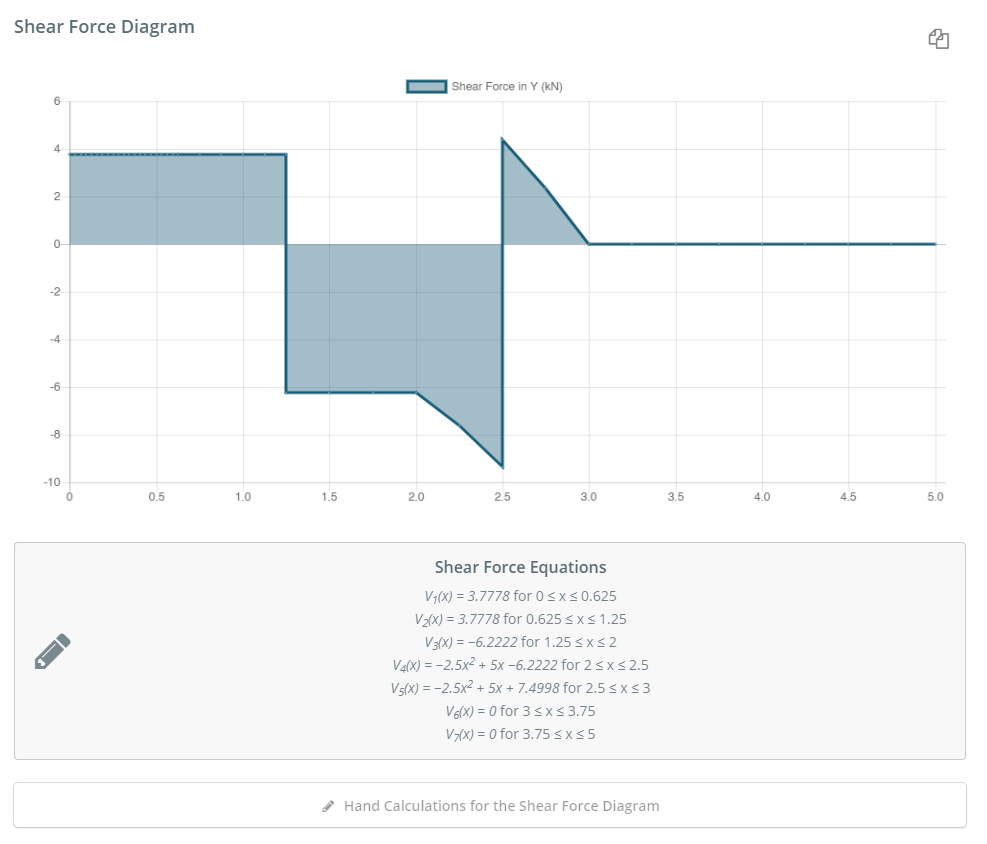## Bending Moment Diagram (BMD)

The Bending Moment Diagram (BMD) of your model is presented with the results. As expected, it plots the bending moment values against the length of the beam.

An option exists to reverse the BMD sign convention. This means that what was considered positive will now be considered negative and vice versa. Click the ‘Reverse BMD Sign Convention’ to toggle this option.

To the right of the BMD, the Bending Moment Equations are presented at each discontinuity. Use the range after the equation to match the equation to its discontinuity on the graph.

To find the value at a specific point on the BMD, use to POI table on the left or hover over the graph to get an exact value at that point.

Hand calculations are also available for the BMD to demonstrate how the Bending Moment Equations were derived. More details about the Hand Calculations are provided in its own section.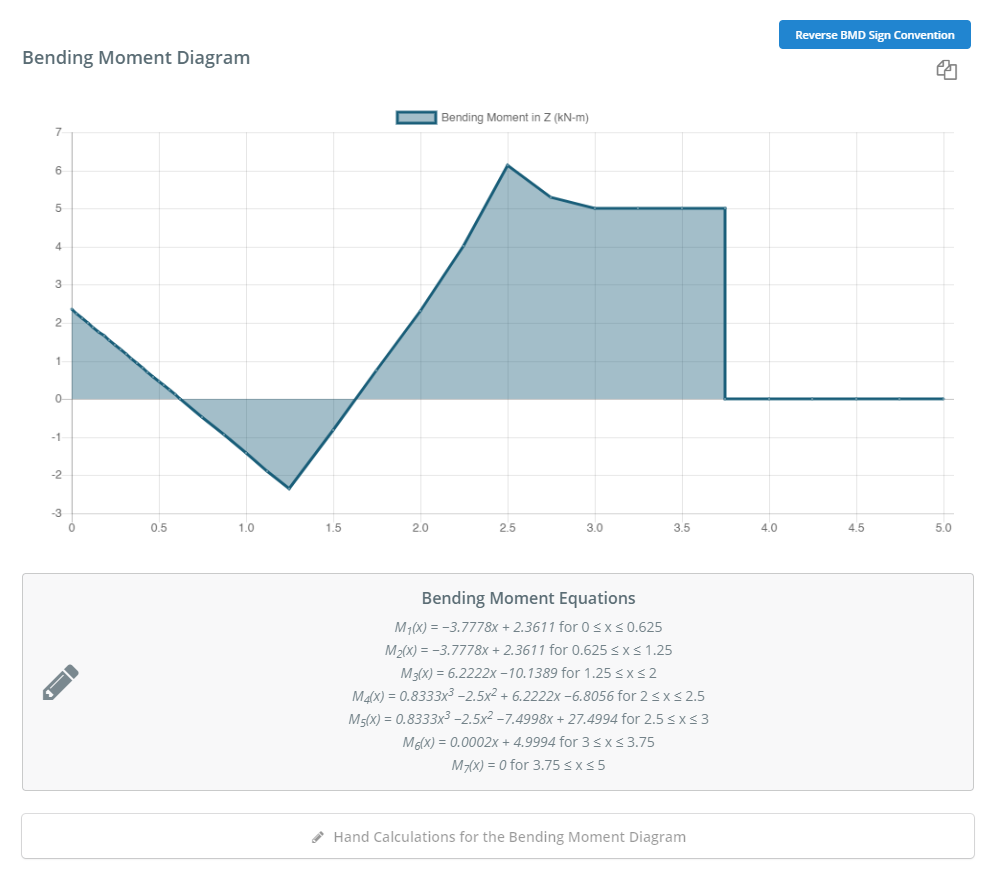## Vertical Deflection and Elastic Curve

The vertical deflection of your model is presented with the results. A diagram showing an elastic curve or deflection of the beam is provided. Below the diagram, a table presenting the minimum and maximum deflection values along the beam is shown. Hand calculations are also available for the Slope and Deflection to demonstrate how the deflection was derived. More details about the Hand Calculations are provided in its own section.

.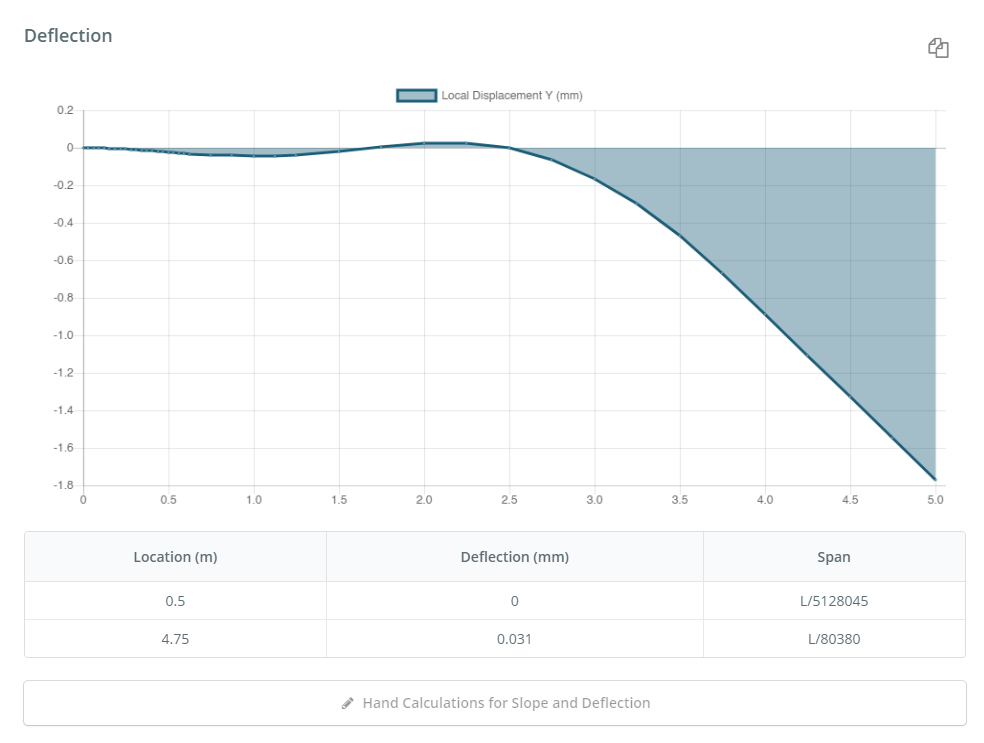## Stress Results

The vertical deflection of your model is presented with the results. A diagram showing an elastic curve or deflection of the beam is provided. Below the diagram, a table presenting the minimum and maximum deflection values along the beam is shown. Hand calculations are also available for the Slope and Deflection to demonstrate how the deflection was derived. More details about the Hand Calculations are provided in its own section.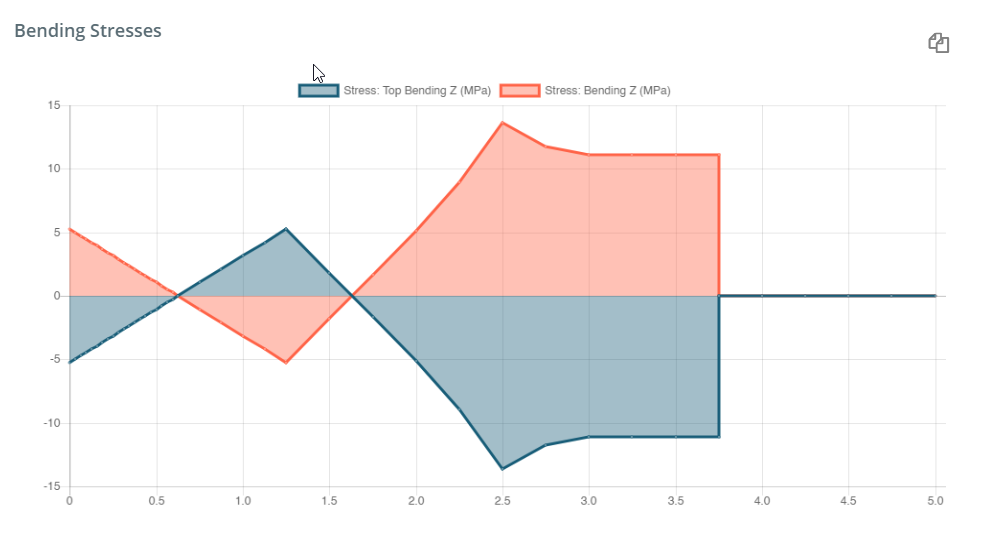## Analysis Summary

The analysis summary tab on the right provides aims to provide a quick summary of the critical results from your beam model. The summary table provides an overview of the deflection, member stress, material yield, and material strength of the beam.

Underneath the Reactions, Force Extremes, and Stress Extremes are listed in table format to provide a quick overview of the critical results of your beam.